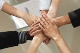GridTop achievementsRank 1
vishal asked on 04 Jul 2014, 12:33 PM
Hello All,

I have activated Avg facility by applying Aggregate="Avg", which works fine but when the column is having no data then also avg counts that and calculates wrong avg.

for example

column1
\$
\$
\$44
\$44
\$44
Avg: \$26.40

Actually it should be \$ 44

How to avoid blanks and How to achieve that?

Thank you!
Vishal

## 3 Answers, 1 is accepted

0Princy
Top achievementsRank 1
answered on 04 Jul 2014, 01:15 PM
Hi Vishal,

This is the default way to calculate average, in-case if you want to avoid the empty rows, you have to write code to handle it and calculate the average. Please take a look at the sample code snippet.

ASPX:
`<``telerik:RadGrid` `ID``=``"RadGrid1"` `runat``=``"server"` `AutoGenerateColumns``=``"false"` `AllowPaging``=``"true"` `OnNeedDataSource``=``"RadGrid1_NeedDataSource"` `ShowFooter``=``"true"` `OnItemDataBound``=``"RadGrid1_ItemDataBound"``>`
`    ``<``MasterTableView``>`
`        ``<``Columns``>`
`            ``<``telerik:GridBoundColumn` `DataField``=``"ID"` `HeaderText``=``"ID"` `UniqueName``=``"ID"` `/>`
`            ``<``telerik:GridBoundColumn` `DataField``=``"Name"` `HeaderText``=``"Name"` `UniqueName``=``"Name"` `/>`
`            ``<``telerik:GridBoundColumn` `DataField``=``"Number"` `HeaderText``=``"Number"` `UniqueName``=``"Number"` `DataFormatString``=``"{0:C}"` `/>`
`        ``</``Columns``>`
`    ``</``MasterTableView``>`
`</``telerik:RadGrid``>`

C#:
`protected` `void` `RadGrid1_NeedDataSource(``object` `sender, GridNeedDataSourceEventArgs e)`
`{`
`    ``dynamic data = ``new``[] {`
`new` `{ ID = 1, Name = ``"Name1"``, Number=0},`
`new` `{ ID = 2, Name = ``"Name2"``, Number=44},`
`new` `{ ID = 3, Name = ``"Name3"``, Number=44},`
`new` `{ ID = 4, Name = ``"Name4"``, Number=0},`
`new` `{ ID = 5, Name = ``"Name5"``, Number=0},`
`new` `{ ID = 6, Name = ``"Name6"``, Number=44},`
`new` `{ ID = 7, Name = ``"Name7"``, Number=44},`
`new` `{ ID = 8, Name = ``"Name8"``, Number=44},`
`new` `{ ID = 9, Name = ``"Name9"``, Number=0}`
`};`
`    ``RadGrid1.DataSource = data;`
`}`
`int` `counter = 0;`
`double` `total = 0, avg = 0; `
`protected` `void` `RadGrid1_ItemDataBound(``object` `sender, GridItemEventArgs e)`
`{       `
`    ``if` `(e.Item ``is` `GridDataItem)`
`    ``{`
`        ``GridDataItem dataItem = (GridDataItem)e.Item;`
`        ``char``[] trimText = { ``'\$'` `};`
`        ``string` `value =(dataItem[``"Number"``].Text).Trim(trimText);`
`        ``Double number = Convert.ToDouble(value);`
`        ``if` `(number != 0)`
`        ``{`
`            ``counter++;`
`            ``total += number;`
`        ``}`
`    ``}`
`    ``if` `(e.Item ``is` `GridFooterItem)`
`    ``{`
`        ``GridFooterItem footerItem = (GridFooterItem)e.Item;           `
`        ``avg = total / counter;`
`        ``footerItem[``"Number"``].Text = ``"Total: \$"` `+ avg.ToString();`
`    ``}`
`}`

Thanks,
Princy
0vishal
Top achievementsRank 1
answered on 08 Jul 2014, 01:19 PM
I tried to do that, but I am not getting correct avg after applying filter.
0Princy
Top achievementsRank 1
answered on 09 Jul 2014, 05:03 AM
Hi Vishal,

Did you try the above sample code snippet? After filtering the grid displays correct average in footer in above example. Can you please elaborate the issue and provide your code or screenshot of your issue. In case if you are having issue in handling Nan values try the following code snippet. You can check this article also to know how to set footers from server side: Totals in Grid Footers

C#:
`if` `(e.Item ``is` `GridFooterItem)`
`{`
` ``GridFooterItem footerItem = (GridFooterItem)e.Item;`
` ``avg = total / counter;`
` ``if` `(avg != 0 && !``double``.IsNaN(avg))`
` ``{`
`  ``footerItem[``"Number"``].Text = ``"Total: \$ "` `+ avg.ToString();`
` ``}`
` ``else`
` ``{`
`  ``footerItem[``"Number"``].Text = ``"Total: \$ 0.0"``;`
` ``}`
`}`

Thanks,
Princy
Tags
Gridvishal
Top achievementsRank 1Rank 1Rank 1